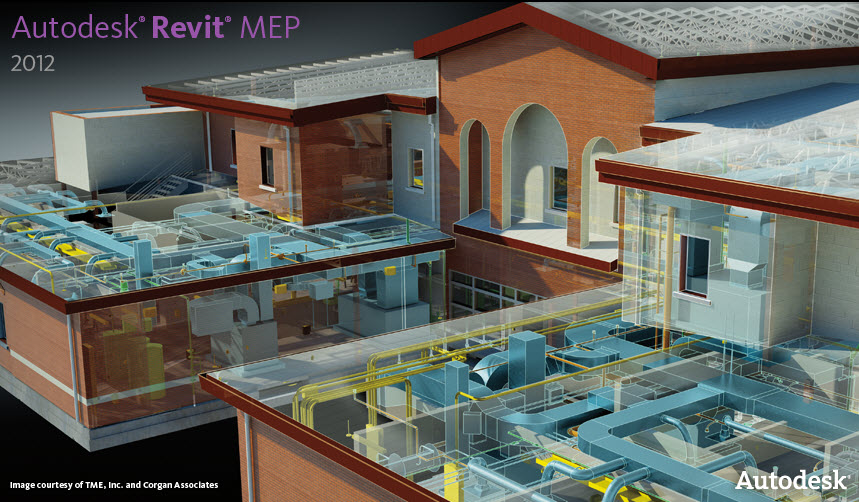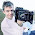## Revit MEP## Friday, October 10, 2008

### Revit Analogy to Reverse Notation Calculators

Reverse notation calculator is a mathematical notation where every operator follows all of its operands. It is also known as Postfix notation and is parenthesis-free.

The Reverse notation scheme was proposed in the early 1960s to reduce computer memory access and utilize the stack to evaluate expressions. The notation and algorithms for this scheme were enriched by Australian philosopher and computer scientist Charles Hamblin in the mid-1960s.

In Reverse notation, the operators follow their operands; for instance, to add three and four, you would write "3 4 +" rather than "3 + 4". If there are multiple operations, the operator is given immediately after its second operand; so the expression written "3 − 4 + 5" in conventional infix notation would be written "3 4 − 5 +" in Reverse notation: first subtract 4 from 3, then add 5 to that. An advantage of Reverse notation is that it obviates the need for parentheses that are required by infix. While "3 − 4 * 5" can also be written "3 − (4 * 5)", that means something quite different from "(3 − 4) * 5", and only the parentheses disambiguate the two meanings. In postfix, the former would be written "3 4 5 * −", which unambiguously means "3 (4 5 *) −".
Interpreters of Reverse notation are often stack-based; that is, operands are pushed onto a stack, and when an operation is performed, its operands are popped from a stack and its result pushed back on. Therefore, Stacks (or Reverse notation), have the advantage of being easy to implement and very fast.
1.